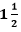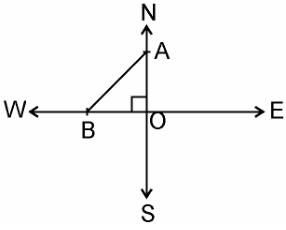Guru

# An aeroplane leaves an airport and flies due north at a speed of 1,000 km per hour. At the same time, another aeroplane leaves the same airport and flies due west at a speed of 1,200 km per hour. How far apart will be the two planes after 1 whole 1/2 hours? Q.11

• 0

I don’t know how to solve this problem form class 10th ncert book of triangles of exercise 6.5 of question no.11 Please give the simple way to solve this problem because it is very tough question An aeroplane leaves an airport and flies due north at a speed of 1,000 km per hour. At the same time, another aeroplane leaves the same airport and flies due west at a speed of 1,200 km per hour. How far apart will be the two planes after 1 whole 1/2 hours?

Share

1. Given,

Speed of first aeroplane = 1000 km/hr

Distance covered by first aeroplane flying due north inhours (OA) = 100 × 3/2 km = 1500 km

Speed of second aeroplane = 1200 km/hr

Distance covered by second aeroplane flying due west inhours (OB) = 1200 × 3/2 km = 1800 kmIn right angle ΔAOB, by Pythagoras Theorem,

AB2 = AO2 + OB2

⇒ AB2 = (1500)2 + (1800)2

⇒ AB = √(2250000 + 3240000)

= √5490000

⇒ AB = 300√61 km

Hence, the distance between two aeroplanes will be 300√61 km.

• 0